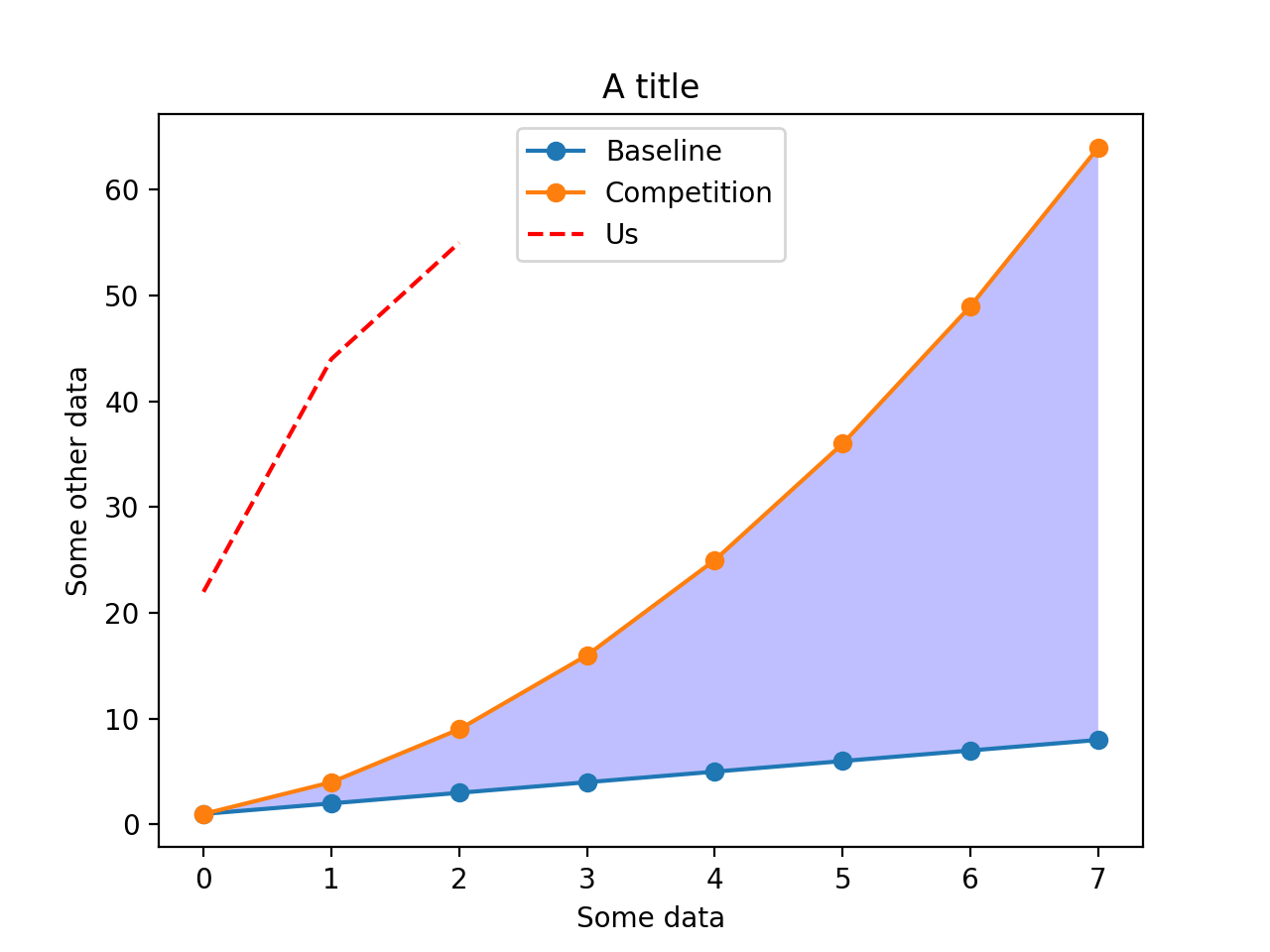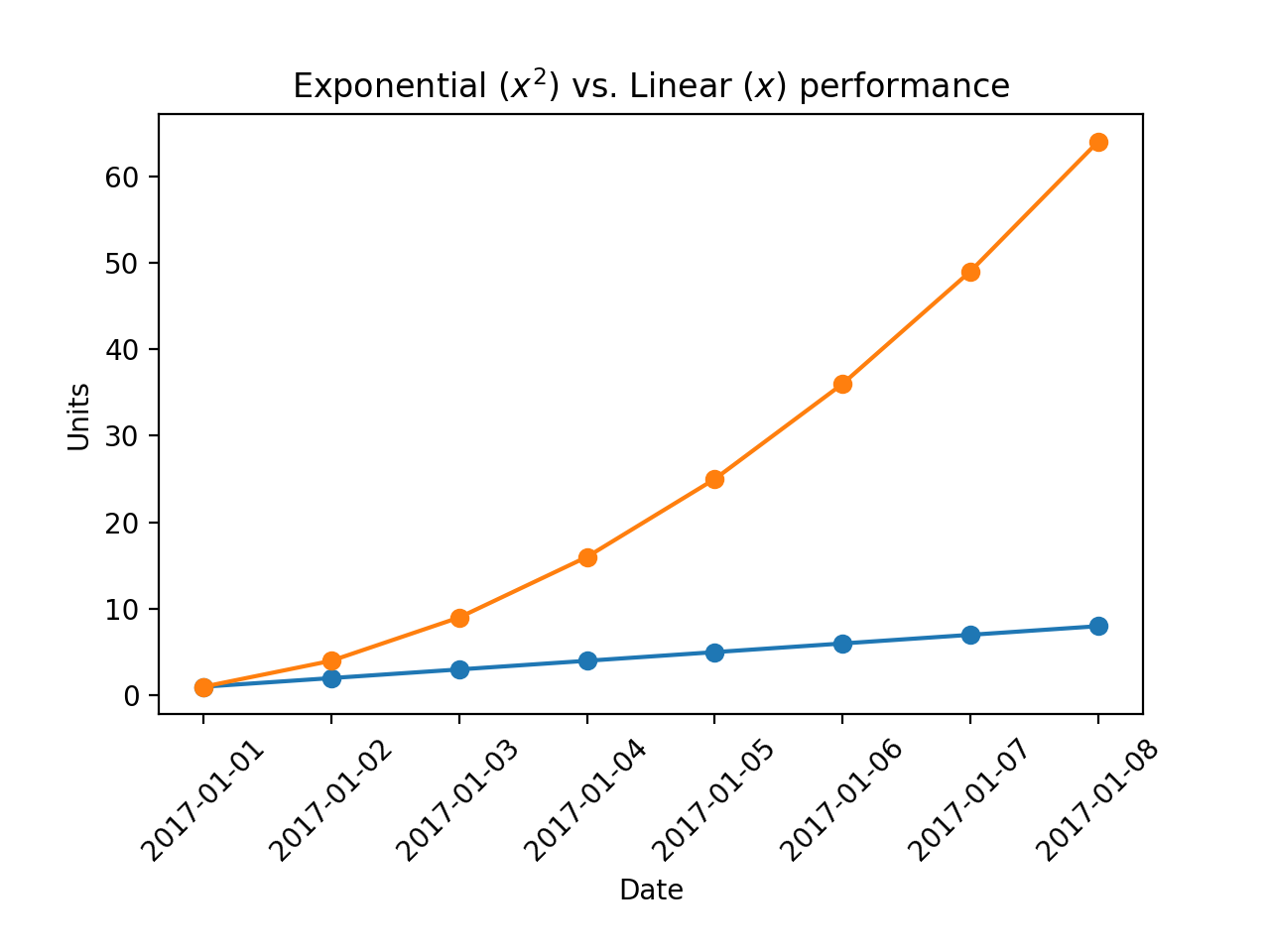# Lineplots

Lineplots are create similarly to scatterplots, except that

• Lineplots infer the index of the data to be the x-axis label. This means that it is often only required to provide the y-dimension.
• Lineplots connect each datapoint with a line.
• Lineplots infer automatically that different series should be labeled differently.
%matplotlib notebook

import matplotlib.pyplot as plt
import numpy as np

linear_data = np.array([1,2,3,4,5,6,7,8])
exponential_data = linear_data**2

plt.figure()
plt.plot(linear_data, '-o',
exponential_data, '-o')
plt.plot([22,44,55], '--r');

<IPython.core.display.Javascript object>Its possible to pass series labels as parameters to the legend function if the series are not initially labeled during plot creation.

plt.xlabel('Some data')
plt.ylabel('Some other data')
plt.title('A title')
plt.legend(['Baseline', 'Competition', 'Us']);


The fill_between function fills an area of a plot.

plt.gca().fill_between(range(len(linear_data)),
linear_data, exponential_data,
facecolor='blue',
alpha=0.25);

plt.figure()

observation_dates = np.arange('2017-01-01', '2017-01-09', dtype='datetime64[D]')

plt.plot(observation_dates, linear_data, '-o',  observation_dates, exponential_data, '-o')

x = plt.gca().xaxis

<IPython.core.display.Javascript object>Rotate text on the x-axis as follows.

for item in x.get_ticklabels():
item.set_rotation(45)


Adjust the subplot so the text doesn’t run off the image.

plt.subplots_adjust(bottom=0.25)


Matplotlib has built-in support for Latex, which allows rendering of equations, such as $x^2$ and $x$.

ax = plt.gca()
ax.set_xlabel('Date')
ax.set_ylabel('Units')
ax.set_title('Exponential vs. Linear performance')
ax.set_title("Exponential ($x^2$) vs. Linear ($x$) performance");